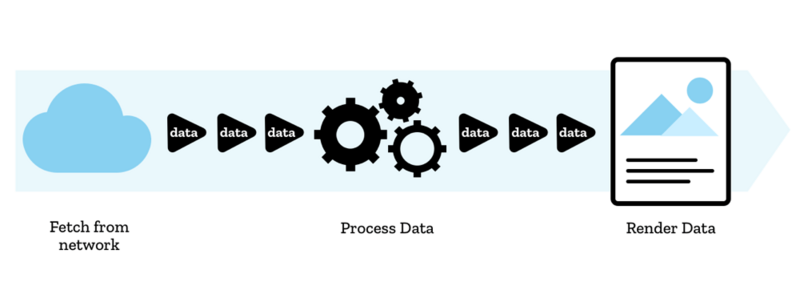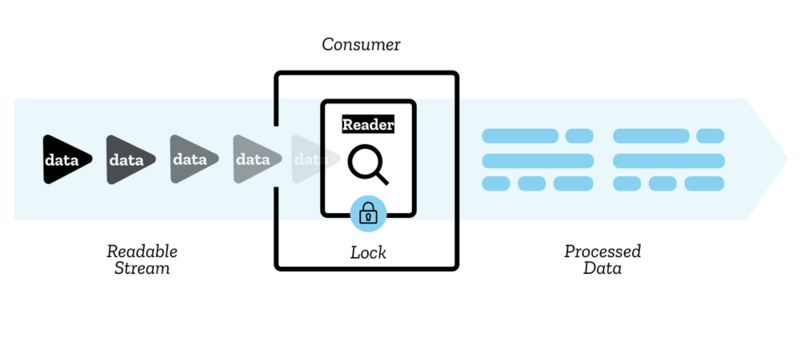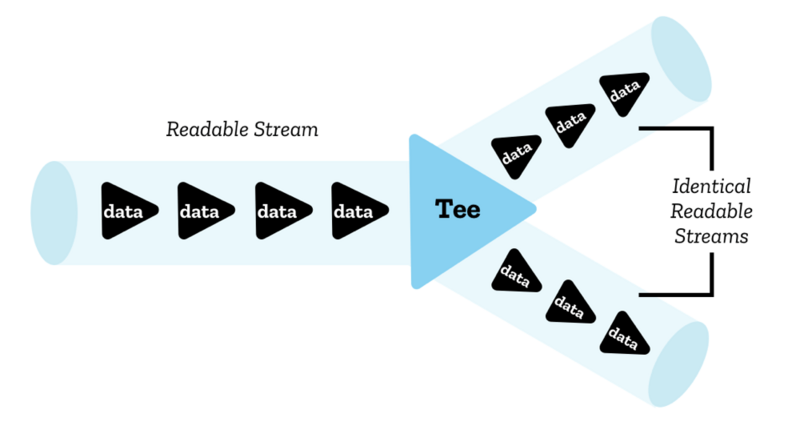# 从 Fetch 到 Streams —— 以流的角度处理网络请求

charlesc

0收藏Fetch API 不香吗？

``````fetch('https://example.org/foo', {
method: 'POST',
mode: 'cors',
'content-type': 'application/json'
},
credentials: 'include',
redirect: 'follow',
body: JSON.stringify({ foo: 'bar' })
}).then(res => res.json()).then(...)``````

``````const res = await fetch('https://example.org/foo', { ... });
const data = await res.json();``````

Fetch API 真香吗？

1. 如何中断一个请求？XMLHttpRequest 对象上有一个 abort() 方法，调用这个方法即可中断一个请求。此外 XHR 还有 onabort 事件，可以监听请求的中断并做出响应。
2. 如何超时中断一个请求？XMLHttpRequest 对象上有一个 timeout 属性，为其赋值后若在指定时间请求还未完成，请求就会自动中断。此外 XHR 还有 ontimeout 事件，可以监听请求的超时中断并做出响应。
3. 如何获取请求的传输进度？

``````const xhr = new XMLHttpRequest();
xhr.open('GET', '/foo');
const { lengthComputable, loaded, total } = event;
if (lengthComputable) {
} else {
}
});
xhr.send();``````

``````const controller = new AbortController();
const { signal } = controller;
fetch('/foo', { signal }).then(...);
signal.onabort = () => { ... };
controller.abort();``````

Streams API 能做什么？

``````const input = fs.createReadStream(null, {
fd, start, end, autoClose: false
});
const output = fs.createWriteStream(outputPath + name);
// 可以从流中直接读取数据
input.on('data', (chunk) => { ... });
// 或者直接将流引向另一个流
input.pipe(zlib.createInflateRaw()).pipe(output);``````Streams API 赋予了网络请求以片段处理数据的能力，过去我们使用 XMLHttpRequest 获取一个文件时，我们必须等待浏览器下载完整的文件，等待浏览器处理成我们需要的格式，收到所有的数据后才能处理它。现在有了流，我们可以以 TypedArray 片段的形式接收一部分二进制数据，然后直接对数据进行处理，这就有点像是浏览器内部接收并处理数据的逻辑。甚至我们可以将一些操作以流的形式封装，再用管道把多个流连接起来，管道的另一端就是最终处理好的数据。

Fetch API 会在发起请求后得到的 Promise 对象中返回一个 Response 对象，而 Response 对象除了提供 headers、redirect() 等参数和方法外，还实现了 Body 这个 mixin 类，而在 Body 上我们才看到我们常用的那些 res.json()、res.text()、res.arrayBuffer() 等方法。在 Body 上还有一个 body 参数，这个 body 参数就是一个 ReadableStream。

ReadableStream• locked
• cancel()
• pipeThrough()
• pipeTo()
• tee()

• closed
• cancel()
• releaseLock()
假设我们需要读取一个流中的的数据，可以循环调用 reader 的 read() 方法，它会返回一个 Promise 对象，在 Promise 中返回一个包含 value 参数和 done 参数的对象。
``````const reader = stream.getReader();
const processData = (result) => {
if (result.done) {
return;
}
const value = result.value; // Uint8Array
const length = value.length;
console.log(`got \${length} bytes data:`, value);
// 读取下一个文件片段，重复处理步骤
};

``````let total = null;
const logProgress = (reader) => {
if (done) {
return;
}
if (total === null) {
} else {
}
});
};
fetch('/foo').then((res) => {
}).then(logProgress);``````

``````let text = '';
const logProcess = (res) => {
const decoder = new TextDecoder('utf-8');
const push = ({ value, done }) => {
if (done) return JSON.parse(text);
text += decoder.decode(value, { stream: true });
// ...
};
};
fetch('/foo').then(logProgress).then((res) => { ... });``````

``````const stream = new ReadableStream({
start(controller) {
// start 方法会在实例创建时立刻执行，并传入一个流控制器
controller.desiredSize
// 填满队列所需字节数
controller.close()
// 关闭当前流
controller.enqueue(chunk)
// 将片段传入流的队列
controller.error(reason)
// 对流触发一个错误
},
pull(controller) {
// 将会在流的队列没有满载时重复调用，直至其达到高水位线
},
cancel(reason) {
// 将会在流将被取消时调用
}
}, queuingStrategy); // { highWaterMark: 1 }``````

``const response = new Response(source, init);``

``````const logProgress = (res) => {
start(controller) {
const push = () => {
if (done) {
controller.close();
return;
}
if (total === null) {
} else {
}
controller.enqueue(value);
push();
});
};
push();
}
});
};
fetch('/foo').then(logProgress).then(res => res.json()).then((data) => { ... });``````所以我们可以利用这个特性将一个流分成两个流，将其中一个流用于输出下载进度，而另一个流直接返回：

``````const logProgress = (res) => {
const [progressStream, returnStream] = res.body.tee();
const log = () => {
if (done) return;
// 省略输出进度
log();
});
};
log();
};
fetch('/foo').then(logProgress).then(res => res.json()).then((data) => { ... });``````

``````let aborter = null;
const abortHandler = (res) => {
start(controller) {
let aborted = false;
const push = () => {
if (done) {
if (!aborted) controller.close();
return;
}
controller.enqueue(value);
push();
});
};
aborter = () => {
controller.error(new Error('Fetch aborted'));
aborted = true;
};
push();
}
});
};
fetch('/foo').then(abortHandler).then(res => res.json()).then((data) => { ... });
aborter();``````

``````let aborter = null;
const abortHandler = (res) => {
aborter = () => res.body.cancel();
return res;
};
fetch('/foo').then(abortHandler).then(res => res.json()).then((data) => { ... });
aborter();``````

``TypeError: Failed to execute 'cancel' on 'ReadableStream': Cannot cancel a locked stream``

``````let aborter = null;
const abortHandler = (res) => {
return res;
};
fetch('/foo').then(abortHandler).then(res => res.json()).then((data) => { ... });
aborter();``````

``TypeError: Failed to execute 'getReader' on 'ReadableStream': ReadableStreamReader constructor can only accept readable streams that are not yet locked to a reader``

``````reader.closed.then(() => {
});

Objects implementing the Body mixin also have an associated consume body algorithm, given a _type_, runs these steps:

If this object is disturbed or locked, return a new promise rejected with a TypeError.
Let stream be body’s stream if body is non-null, or an empty ReadableStream object otherwise.
Let reader be the result of getting a reader from _stream_. If that threw an exception, return a new promise rejected with that exception.
Let promise be the result of reading all bytes from stream with _reader_.
Return the result of transforming promise by a fulfillment handler that returns the result of the package data algorithm with its first argument, type and this object’s MIME type.

• 发起请求
• 从响应头中拿到 Content-Length 属性
• 在响应过程中拿到正在下载的数据
• 终止下载
• 重新下载，但是此时根据已经拿到的数据设置 Range 请求头
• 重复步骤 3-5，直至下载完成
• 下载完成，将已拿到的数据拼接成完整的

``````let chunks = [];
const xhr = new XMLHttpRequest();
xhr.open('GET', '/foo');
xhr.responseType = 'moz-chunked-arraybuffer';
chunks.push(xhr.response);
});
const blob = new Blob(chunks);
});
xhr.send();``````

``````const chunks = [];
let length = 0;
const chunkCache = (res) => {
start(controller) {
const push = () => {
if (done) {
let chunk;
while (chunk = chunks.shift()) {
controller.enqueue(chunk);
}
controller.close();
return;
}
chunks.push(value);
length += value.length;
push();
});
};
push();
}
});
};
const controller = new AbortController();
fetch('/foo', {
'Range': `bytes=\${length}-`
},
signal: controller.signal
}).then(chunkCache).then(...);
// 请求中断后再次执行上述 fetch() 方法``````帖子
视频
声望
粉丝
社区精华内容